# ML Aggarwal Class 8 Solutions for ICSE Maths Chapter 9 Direct and Inverse Variation Objective Type Questions

## ML Aggarwal Class 8 Solutions for ICSE Maths Chapter 9 Direct and Inverse Variation Objective Type Questions

Mental Maths
Question 1.
Fill in the blanks:
(i) Two quantities are said to be in direct variation if the increase (or decrease) in one quantity causes in ………….. other quantity.
(ii) Two quantities X and Y are said to be in inverse variation if XY is …………..
(iii) The total cost of articles varies ………….. to the number of articles purchased.
(iv) More work is done in ………….. time.
(v) The time taken to finish work varies ………….. to the number of men at work.
(vi) The speed of a moving object varies inversely to the ………….. to cover a certain distance.
(vii) The number of articles varies ………….. with the cost per article if a fixed amount is available.
(viii)Remuneration is in ………….. of work done.
Solution: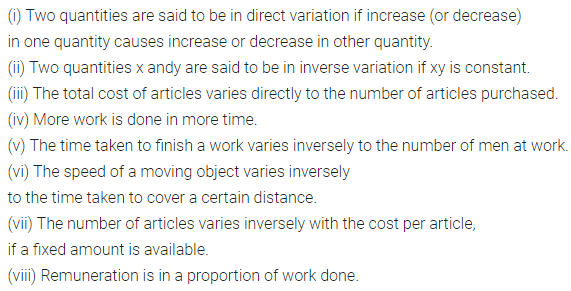Question 2.
State whether the following statements are true (T) or false (F):
(i) Two quantities x andy are said to be in inverse variation if $$\frac{x}{y}$$ is constant.
(ii) Number of days needed to complete the work = $$\frac{1}{\text { one day’s work }}$$
(iii) Two quantities x andy are said to be in direct variation if x = ky, where k is constant of variation.
(iv) The work done varies inversely to the number of men at work.
(v) In the given time, the distance covered by a moving object varies directly to its speed.
(vi) If A can complete a work in n days, then A’s one day’s work is $$\frac{1}{n}$$ of the work, n
(vii) More the money deposited in a bank, more is the interest earned.
(viii) If the number of articles purchased increases the total cost decreases.
(ix) At the same time length of shadow is in direct variation with length of the object.
(x) The distance covered varies inversely to the consumption of petrol.
Solution: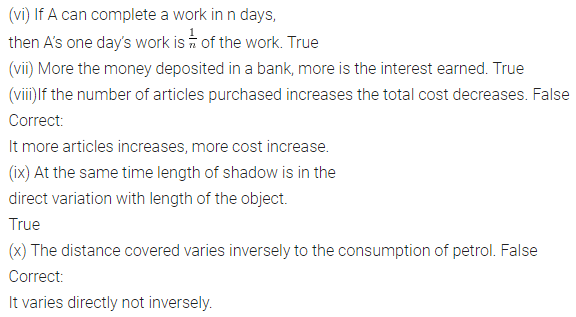Multiple Choice Questions
Choose the correct answer from the given four options (3 to 13):
Question 3.
Two quantities x and y are said to be in inverse variation if
(a) xy = k
(b) x ∝ $$\frac{1}{y}$$
(c) x = $$\frac{k}{y}$$
(d) all of these
Solution: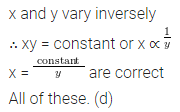Question 4.
If 12-metre wire costs ₹24, then the cost of 8-metre wire is
(a) ₹16
(b) ₹20
(c) ₹12
(d) ₹18
Solution:Question 5.
If 5 kg wheat cost ₹60, then cost of 20 kg wheat is
(a) ₹200
(b) ₹210
(c) ₹220
(d) ₹240
Solution:Question 6.
If 10-men can complete a work in 6 days, then 30 men can complete the same work in
(a) 2 days
(b) 3 days
(c) 4 days
(d) 5 days
Solution:Question 7.
A car travels 80 km in 5 litres of petrol, then the distance covered by it in 15 litres of petrol is
(a) 400 km
(b) 240 km
(c) 200 km
(d) 100 km
Solution: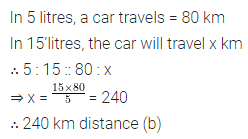Question 8.
In a mess, there was enough food for 200 students for 20 days. If 50 new students joined them, then the food will last for
(a) 15 days
(b) 16 days
(c) 17 days
(d) 18 days
Solution:Question 9.
3 persons can paint a house in 8 days, then 4 persons can paint the same house in
(a) 5 days
(b) 6 days
(c) 7 days
(d) none of these
Solution: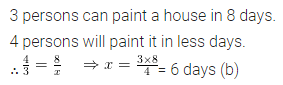Question 10.
A photograph of bacteria is enlarged 100000 times attains a length of 5 cm, then actual length of the bacteria is
(a) 0.00005 cm
(b) 5 × 10-5
(c) 5 × 10-7
(d) all of these
Solution: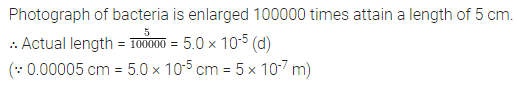Question 11.
A tree 12 metre high casts a shadow of length 8 metre. Height of the tree whose shadow is 6 metre in length is
(a) 6 m
(b) 9 m
(c) 15 m
(d) none of these
Solution: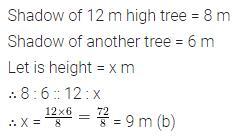Question 12.
If 5 pipes can fill the tank in 1 hour, then 4 pipes will fill the tank in
(a) 75 minutes
(b) 70 minutes
(c) 65 minutes
(d) none of these
Solution: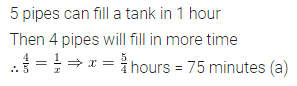Question 13.
A tap fills a tank in 8 hours and another tap at the bottom empties it in 10 hours. If both work together, the tank will be filled in
(a) 18 hours
(b) 24 hours
(c) 36 hours
(d) 40 hours
Solution:Value-Based Questions
Question 1.
The cost of fuel for running a train is proportional to the speed generated in km/h. It costs ₹40 per hour when train is moving with 20 km/h. What would be the cost of fuel per hour, if the train is moving with 60 km/h?
Keeping the safety and fuel prices in mind, state the values promoted in the question.
Solution:Question 2.
A pipe can fill a tank in 9 hours. There is a leakage in the bottom of the tank due to which tank is filled in 12 hours. If the tank is full, how much time will leakage take to empty the tank? Should we repair the leakage tank? Should we repair the leakage of the tank immediately? What values are being promoted?
Solution:Higher Order Thinking Skills (Hots)
Question 1.
If 8 labourers can earn ₹9000 in 15 days, how many labourers can earn ₹6300 in 7 days?
Solution:Question 2.
Three typists working 8 hours a day type a document in 10 days. If only 2 typists are working, how many hours a day should they work to finish the job in 12 days?
Solution: